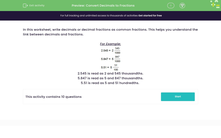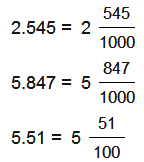# Convert Decimals to Fractions

In this worksheet, students practise changing decimal numbers to their fraction equivalents.Key stage:  KS 2

Curriculum topic:   Number: Fractions, Decimals and Percentages

Curriculum subtopic:   Calculate Fraction-Decimal Equivalents

Popular topics:   Fractions worksheets, Percentages worksheets, Decimals worksheets

Difficulty level:#### Worksheet Overview

In this worksheet, write decimals or decimal fractions as common fractions. This helps you understand the link between decimals and fractions.

For Example:2.545 is read as 2 and 545 thousandths.

5.847 is read as 5 and 847 thousandths.

5.51 is read as 5 and 51 hundredths.

### What is EdPlace?

We're your National Curriculum aligned online education content provider helping each child succeed in English, maths and science from year 1 to GCSE. With an EdPlace account you’ll be able to track and measure progress, helping each child achieve their best. We build confidence and attainment by personalising each child’s learning at a level that suits them.

Get started